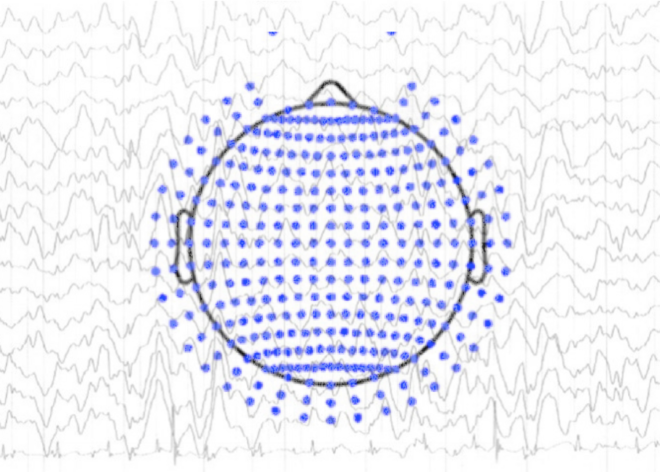# Lab Talk# Common Average vs Infinity Reference in EEG

How do the average and infinity references in EEG compare, and what are other reference free approaches?

In the previous blogpost, we looked at how the choice of reference impacts EEG power spectral analysis and connectivity estimation. In this blogpost, we will compare the two commonly used reference techniques – average reference and infinity reference in more detail and also look at other reference-free approaches.

There was previously widespread consensus in the EEG community to re-reference EEG data to a common average reference. However recently this notion has been challenged by the infinity reference.

### The Average Reference

To recap, the idea behind average reference is to compute the average of the signal at all EEG electrodes and subtract it from the EEG signal at every electrode for every time point. The simple rationale behind this is that we can approximate the head as a sphere and assuming that the sum of all the potentials recorded on the whole sphere due to current sources inside it is zero, this would make for a quiet or electrically neutral reference.  However, in practice, to achieve such an ideal reference one would require large number of electrodes that cover the whole head uniformly, which is not the case in EEG recordings where limited number of electrodes cover mostly the upper part of the head .

### Infinity Reference or Reference Electrode Standardization Technique (REST)

To mitigate this issue Yao and colleagues introduce the reference electrode standardization technique (REST) or infinity reference technique, where the idea is to come up with a virtual reference location that is at infinity .  The idea of REST is based on the assumption that the same underlying sources S are responsible for the EEG data recorded with two different references.  Using a linear relationship, EEG data recoded with an infinity reference can be expressed as

V = GS

where G is the lead-filed matrix or simply a transfer matrix which maps the sources S to the EEG sensors. Such a matrix is typically obtained by constructing the head model. Let’s assume that we have recorded EEG data, Vm with reference to mastoid, which can be expressed as

Vm = Gm S

Where Gm is the lead-field matrix (similar to G but with mastoid reference). Combining the above two equations we have,

V =G*(Gm+*Vm )

where Gm+ is the pseudo-inverse of  Gm.  Thus in order to re-reference EEG data to infinity reference, one simply needs to compute G*Gm+.  Although a simple approach, REST depends fundamentally on the head model, which can lead to biased estimates due to uncertainty regarding tissue conductivity and inaccurate segmentation.  Thus, although REST can also be affected by electrode coverage, having an accurate head model is the most important factor, since accurate head model can decrease coverage error .

### Other reference-free approaches

Commonly used in clinical settings, the bipolar EEG is obtained by subtracting the potentials of two neighboring EEG electrodes. This has an effect of removing all signals common to the two electrodes, including the common reference. Although bipolar recordings give more ‘localized picture’ of electrical activity, which is useful in for examples, cases of epilepsy, the assumption that all signals common to the two electrodes are from the reference is incorrect, and it can result in missing genuine underlying synchrony as well as dipoles with certain locations and orientations. Thus the use of bipolar EEG precludes effective coherence or connectivity analysis.

By making use of spline-interpolation, the surface laplacian can be computed for scalp EEG, which is basically the spatial (second) derivative of the EEG potential .  The surface laplacian is not biased by the reference choice. However, it has a disadvantage of being biased to shallow and localized sources and missing distributed sources.

Finally, instead of computing connectivity on scalp-EEG data, one can perform such estimation on the source level by solving the EEG inverse problem, which is shown not to be affected by the choice of the reference. The REST approach seems to deliver connectivity results that are least affected by the reference compared to other reference techniques, at least as shown under simulated circumstances. However, the downside of this approach is that it depends on accurate head models / conductivity value for the tissues in order to obtain reliable estimates of the underlying sources.

Using reference-free techniques like bipolar derivations, surface Laplacian or source estimation have their set of own assumptions and challenges which can also bias the results.  In conclusion, when analyzing and interpreting scalp-EEG data, the choice of reference has to be taken into account.  Ultimately there is no perfect solution and outcomes need to be considered in a reference specific manner.

### References

1. Nunez, Paul L. “REST: a good idea but not the gold standard.” Clinical neurophysiology: official journal of the International Federation of Clinical Neurophysiology12 (2010): 2177.
2. Yao, D. (2001). A method to standardize a reference of scalp EEG recordings to a point at infinity. Physiol. Meas. 22, 693–711. doi:10.1088/0967-3334/22/4/305
3. Nunez, Paul L., and Ramesh Srinivasan. Electric fields of the brain: the neurophysics of EEG. Oxford University Press, USA, 2006.

### One thought on “Common Average vs Infinity Reference in EEG”

1.André Achim says:

An alternate choice is the Balanced Non-Cephalic Reference of Stephenson & Gibbs (1951): https://www.sciencedirect.com/science/article/abs/pii/001346945190017X
In this, electrodes around the neck pick up opposite versions of the EKG and a potentiometer finds the best annihilation of their EKG signals.
In the REST approach, if say Gm is 128 channels x 2000 possible source positions, the pseudo-inverse of Gm involves inverting a 2000 x 2000 matrix that has effective rank 128. I wonder how this could be reliable.